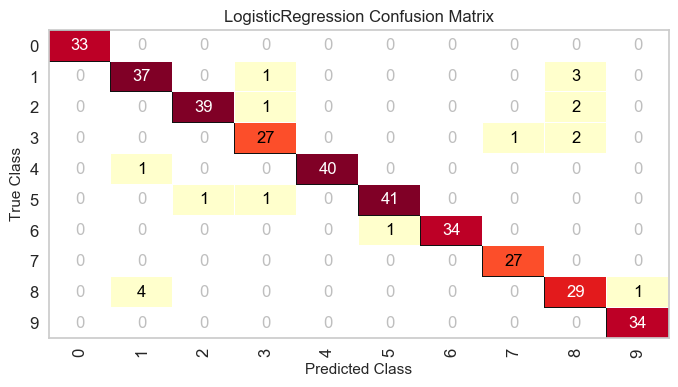# Confusion Matrix¶

The ConfusionMatrix visualizer is a ScoreVisualizer that takes a fitted scikit-learn classifier and a set of test X and y values and returns a report showing how each of the test values predicted classes compare to their actual classes. Data scientists use confusion matrices to understand which classes are most easily confused. These provide similar information as what is available in a ClassificationReport, but rather than top-level scores, they provide deeper insight into the classification of individual data points.

Below are a few examples of using the ConfusionMatrix visualizer; more information can be found by looking at the scikit-learn documentation on confusion matrices.

from sklearn.datasets import load_digits
from sklearn.model_selection import train_test_split
from sklearn.linear_model import LogisticRegression

from yellowbrick.classifier import ConfusionMatrix

# We'll use the handwritten digits data set from scikit-learn.
# Each feature of this dataset is an 8x8 pixel image of a handwritten number.
# Digits.data converts these 64 pixels into a single array of features
X = digits.data
y = digits.target

X_train, X_test, y_train, y_test = train_test_split(X, y, test_size =0.2, random_state=11)

model = LogisticRegression()

# The ConfusionMatrix visualizer taxes a model
cm = ConfusionMatrix(model, classes=[0,1,2,3,4,5,6,7,8,9])

# Fit fits the passed model. This is unnecessary if you pass the visualizer a pre-fitted model
cm.fit(X_train, y_train)

# To create the ConfusionMatrix, we need some test data. Score runs predict() on the data
# and then creates the confusion_matrix from scikit-learn.
cm.score(X_test, y_test)

# How did we do?
cm.poof()## Plotting with Class Names¶

Class names can be added to a ConfusionMatrix plot using the label_encoder argument. The label_encoder can be a sklearn.preprocessing.LabelEncoder (or anything with an inverse_transform method that performs the mapping), or a dict with the encoding-to-string mapping as in the example below:

iris = load_iris()
X = iris.data
y = iris.target
classes = iris.target_names

X_train, X_test, y_train, y_test = tts(X, y, test_size=0.2)

model = LogisticRegression()

iris_cm = ConfusionMatrix(
model, classes=classes,
label_encoder={0: 'setosa', 1: 'versicolor', 2: 'virginica'}
)

iris_cm.fit(X_train, y_train)
iris_cm.score(X_test, y_test)

iris_cm.poof()### API Reference¶

Visual confusion matrix for classifier scoring.

class yellowbrick.classifier.confusion_matrix.ConfusionMatrix(model, ax=None, classes=None, sample_weight=None, percent=False, label_encoder=None, cmap='YlOrRd', fontsize=None, **kwargs)[source]

Bases: yellowbrick.classifier.base.ClassificationScoreVisualizer

Creates a heatmap visualization of the sklearn.metrics.confusion_matrix(). A confusion matrix shows each combination of the true and predicted classes for a test data set.

The default color map uses a yellow/orange/red color scale. The user can choose between displaying values as the percent of true (cell value divided by sum of row) or as direct counts. If percent of true mode is selected, 100% accurate predictions are highlighted in green.

Requires a classification model.

Parameters: model : estimator Must be a classifier, otherwise raises YellowbrickTypeError ax : matplotlib Axes, default: None The axes to plot the figure on. If None is passed in the current axes will be used (or generated if required). sample_weight: array-like of shape = [n_samples], optional Passed to confusion_matrix to weight the samples. percent: bool, default: False Determines whether or not the confusion_matrix is displayed as counts or as a percent of true predictions. Note, if specifying a subset of classes, percent should be set to False or inaccurate figures will be displayed. classes : list, default: None a list of class names to use in the confusion_matrix. This is passed to the labels parameter of sklearn.metrics.confusion_matrix(), and follows the behaviour indicated by that function. It may be used to reorder or select a subset of labels. If None, classes that appear at least once in y_true or y_pred are used in sorted order. label_encoder : dict or LabelEncoder, default: None When specifying the classes argument, the input to fit() and score() must match the expected labels. If the X and y datasets have been encoded prior to training and the labels must be preserved for the visualization, use this argument to provide a mapping from the encoded class to the correct label. Because typically a Scikit-Learn LabelEncoder is used to perform this operation, you may provide it directly to the class to utilize its fitted encoding. cmap : string, default: 'YlOrRd' Specify a colormap to define the heatmap of the predicted class against the actual class in the confusion matrix. fontsize : int, default: None Specify the fontsize of the text in the grid and labels to make the matrix a bit easier to read. Uses rcParams font size by default.

Examples

>>> from yellowbrick.classifier import ConfusionMatrix
>>> from sklearn.linear_model import LogisticRegression
>>> viz = ConfusionMatrix(LogisticRegression())
>>> viz.fit(X_train, y_train)
>>> viz.score(X_test, y_test)
>>> viz.poof()

Attributes: score_ : float Global accuracy score confusion_matrix_ : array, shape = [n_classes, n_classes] The numeric scores of the confusion matrix class_counts_ : array, shape = [n_classes,] The total number of each class supporting the confusion matrix
draw()[source]

Renders the classification report; must be called after score.

finalize(**kwargs)[source]

Finalize executes any subclass-specific axes finalization steps.

Parameters: kwargs: dict generic keyword arguments.

Notes

The user calls poof and poof calls finalize. Developers should implement visualizer-specific finalization methods like setting titles or axes labels, etc.

score(X, y)[source]

Draws a confusion matrix based on the test data supplied by comparing predictions on instances X with the true values specified by the target vector y.

Parameters: X : ndarray or DataFrame of shape n x m A matrix of n instances with m features y : ndarray or Series of length n An array or series of target or class values score_ : float Global accuracy score